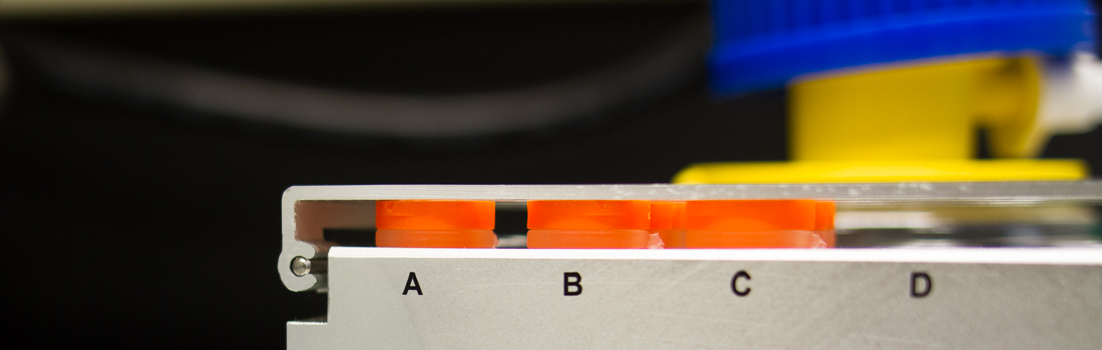# Biosensor Core# Establishing Optimal Conditions to Measure Binding

The optimal conditions for studies of binding are determined by the kind of study we are doing. In Yes-No binding, as just illustrated, we typically capture large amounts of the ligand on the chip surface in order to optimize our chance of detecting binding when we flow the analyte solution over the surface. For studies of high affinity binding, typically much lower amounts of ligand should be captured on the surface, to avoid artifacts associated with a phenomenon called “Mass Transfer” and to optimize the accuracy of curve fitting (“Evaluating the Data“).

A key concept in establishing conditions for binding studies is Rmax. Rmax is the rough equivalent of Bmax in other binding studies, i.e., the maximum amount of binding obtainable in your reaction. For example, if you have 100 nMoles of an antibody, it should have a Bmax for its antigen of 200 nMoles, assuming both of the sites on the antibody bind antigen independently. For Biacore® studies, rather than calculating maximum binding on the basis of the amount of the ligand and analyte in solution (e.g., 100 nMoles in 1 ml), the maximum signal due to binding, Rmax, is calculated from the number of RUs of ligand captured on the chip, the relative molecular masses of the captured ligand and the analyte of interest, and the number of binding sites on the captured ligand that can bind analyte. The formula for Rmax is therefore

Rmax = n•RUL•MA•ML-1

where n is the number of binding sites per ligand molecule, RUL is the number of RU of ligand captured on the chip, MA is the molecular mass of the analyte, and ML is the molecular mass of the ligand. Thus, for the example given above, assuming a divalent antibody (ligand) with a molecular mass (Mr) =150 kDa, an antigen (analyte) with Mr = 100 kDa, and an initial capture of antibody to 100 RUs,

Rmax = 2•100RU•100kDa/150kDa  = 133 RU

In another example, in which the ligand, a GST fusion protein, Mr = 40 kDa, is captured on the chip to 80 RUs, the analyte is an MBP fusion protein, Mr = 60 kDa, and binding is 1:1,

Rmax = 1•80RU•60kDa/40kDa  = 120 RU

Rmax values should be calculated for each experiment, both because they help set the optimal conditions for binding, and because they can be used to determine how much of the protein captured directly or indirectly on the chip surface is active. Biacore® software reveals what the actual Rmax for any set of measurements really is. Thus, if in the second example the calculation indicates that the actual Rmax is only 60 RU, it would indicate that 50% of the GST fusion protein bound to the chip surface is inactive. This is an important feature of Biacore® measurements that is not readily available from other, less quantitative methods used to study binding.